# Иностранная литература и современность: General Statistics: Ch 6 Quiz Flashcards

Set Details Share
created 9 years ago by GreenHero64
76,725 views
This course is designed to acquaint the student with the principles of descriptive and inferential statistics. Topics will include: types of data, frequency distributions and histograms, measures of central tendency, measures of variation, probability, probability distributions including binomial, normal probability and student's t distributions, standard scores, confidence intervals, hypothesis testing, correlation, and linear regression analysis. This course is open to any student interested in general statistics and it will include applications pertaining to students majoring in athletic training, pre-nursing and business.
updated 9 years ago by GreenHero64
College: First year, College: Second year, College: Third year, College: Fourth year
Subjects:
statistics, mathematics, probability & statistics
Page to share:
Embed this setcancel
COPY
code changes based on your size selection
Size:
X

1

Assume that thermometer readings are normally distributed with a mean of 0°C and a standard deviation of 1.00°C. A thermometer is randomly selected and tested.

For the case​ below, draw a​ sketch, and find the probability of the reading.​ (The given values are in Celsius​ degrees.)

Between –1.47 and 1.87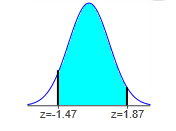The probability of getting a reading between –1.47°C and 1.87°C is 0.8985 .

P(-1.47 < z < 1.87)

= (0.9693 – 0.0708)

2

Assume the readings on thermometers are normally distributed with a mean of 0°C and a standard deviation of 1.00°C.

Find the probability that a randomly selected thermometer reads greater than 0.04 and draw a sketch of the region.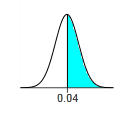The probability is 0.4840.

P(z > 0.04)

= 1 – P(z < 0.04)

= 1 – 0.5160 = 0.4840

OR

Symmetric to z score of -0.04 (Area = 0.4840)

3

Assume that a randomly selected subject is given a bone density test. Those test scores are normally distributed with a mean of 0 and a standard deviation of 1.

Find the probability that a given score is less than –0.56 and draw a sketch of the region.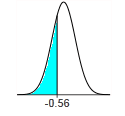The probability is 0.2877.

P(z < -0.56) = 0.2877

4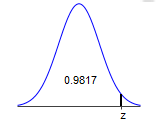Find the indicated z score. The graph depicts the standard normal distribution with mean 0 and standard deviation 1.

The indicated z score is 2.09.

5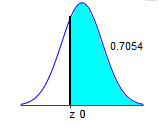Find the indicated z score. The graph depicts the standard normal distribution with mean 0 and standard deviation 1.

The indicated z score is –0.54.

Symmetric to Area of 0.7054 (z = 0.54)

OR

Area = 1 – 0.7054 = 0.2946

6

A statistics professor plans classes so carefully that the lengths of her classes are uniformly distributed between 49.0 and 59.0 minutes.

Find the probability that a given class period runs between 50.5 and 51.5 minutes.

0. 1 00

49.0 = 10.0

Since the uniform distribution is​ rectangular, has a length of 10.0​, and an area of​ 1, the height of the uniform distribution is 0.1.

P(between 50.5 and 51.5​) = ​(shaded region ​length) × (shaded region​ height)

= ​(51.5 – 50.5​) × (0.1​)

= 0.1

7

Assume that a randomly selected subject is given a bone density test. Those test scores are normally distributed with a mean of 0 and a standard deviation of 1.

Find the probability that a given score is less than 3.69 and draw a sketch of the region.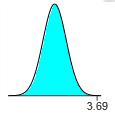The probability is 0.9999.

8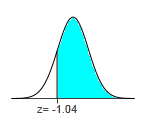Find the area of the shaded region. The graph depicts the standard normal distribution of bone density scores with mean 0 and standard deviation 1.

The area of the shaded region is 0.8508.

P(z > 0.04)

= 1 – P(z < -1.04)

= 1 – 0.1492 = 0.8505

OR

Symmetric to z score of 1.04 (Area = 0.8508)

9

The waiting times between a subway departure schedule and the arrival of a passenger are uniformly distributed between 0 and 6 minutes. Find the probability that a randomly selected passenger has a waiting time greater than 4.25 minutes.

0.298

P(greater than 45.25​) = ​(length of shaded ​region) ​× (height of shaded​ region)

= ​(6 – 5.25​) × ​(0.167)-round to 2 dec. places

= ​(1.75​ × (0.17)

= 0.2975

10

Assume that thermometer readings are normally distributed with a mean of 0°C and a standard deviation of 1.00°C. A thermometer is randomly selected and tested.

For the case​ below, draw a​ sketch, and find the probability of the reading.​ (The given values are in Celsius​ degrees.)

Between –0.50 and 2.00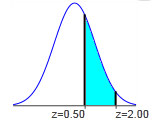The probability of getting a reading between 0.50°C and 2.00°C is 0.2857.

P(0.50 < z < 2.00)

= (0.9772 – 0.6915)